Students can download Maths Chapter 8 Statistics Ex 8.3 Questions and Answers, Notes, Samacheer Kalvi 9th Maths Guide Pdf helps you to revise the complete Tamilnadu State Board New Syllabus, helps students complete homework assignments and to score high marks in board exams.

## Tamilnadu Samacheer Kalvi 9th Maths Solutions Chapter 8 Statistics Ex 8.3

Question 1.
The monthly salary of 10 employees in a factory are given below:
Rs 5000, Rs 7000, Rs 5000, Rs 7000, Rs 8000, Rs 7000, Rs 7000, Rs 8000, Rs 7000, Rs 5000
Find the mean, median and mode.
Solution: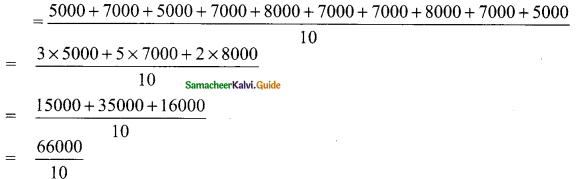Mean = 6600
Median:
Arrange in ascending order we get.
5000, 5000, 5000, 7000, 7000, 7000, 7000, 7000, 8000, 8000
The number of values = 10
Median = Average of ($$\frac{10}{2})^{th}$$ and ($$\frac{10}{2}+1)^{th}$$
= Average of 5th value and 6th value
= $$\frac{7000+7000}{2}$$
∴ Median = 7000
Mode: 7000 repeated 5 times
∴ Mode = 7000

Question 2.
Find the mode of the given data: 3.1, 3.2, 3.3, 2.1, 1.3, 3.3, 3.1
Solution:
3.1 occuring two times
3.3 occuring two times
∴ 3.1 and 3.3 are the mode (bimodal)Question 3.
For the data 11, 15, 17, x + 1, 19, x – 2, 3 if the mean is 14, find the value of x. Also find the mode of the data.
Solution:
Arithmetic mean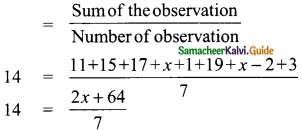∴ 2x + 64 = 14 × 7
2x = 98 – 64
2x = 34
x = $$\frac{34}{2}$$
= 17
The given numbers are 11, 15, 17, 18, 19, 15 and 3
15 occuring two times
∴ Mode = 15
The value of x = 17 and mode = 15Question 4.
The demand of track suit of different sizes as obtained by a survey is given below: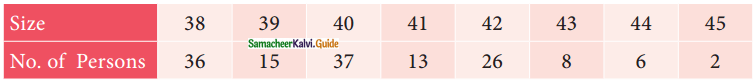Which size is demanded more?
Solution:
The highest frequency is 37
The corresponding value is the mode
∴ Mode = 40
Size 40 is demanded more.Question 5.
Find the mode of the following data: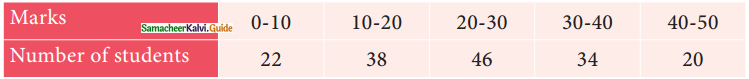Solution: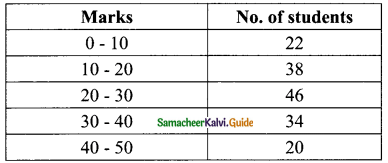The highest frequency is 46
20 – 30 is the modal class
Here l = 20, f = 46, f1 = 38, f2 = 34 and c = 10
Mode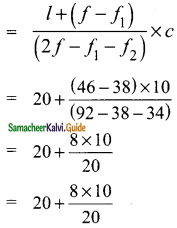= 20 + 4
= 24
∴ Mode = 24Question 6.
Find the mode of the following distribution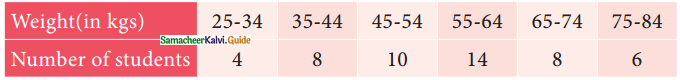Solution:
In the given table the class intervals are in inclusive form; convert them into exclusive form.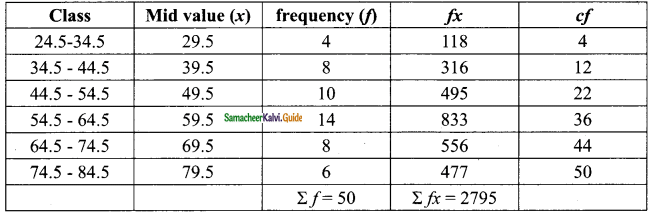The highest frequency is 14
Modal class is 54.5 – 64.5
Here l = 54.5, f = 14, f1 = 10, f2 = 8 and c = 10
mode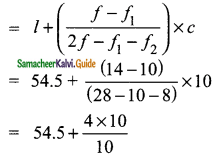= 58.5
∴ Mode = 58.5